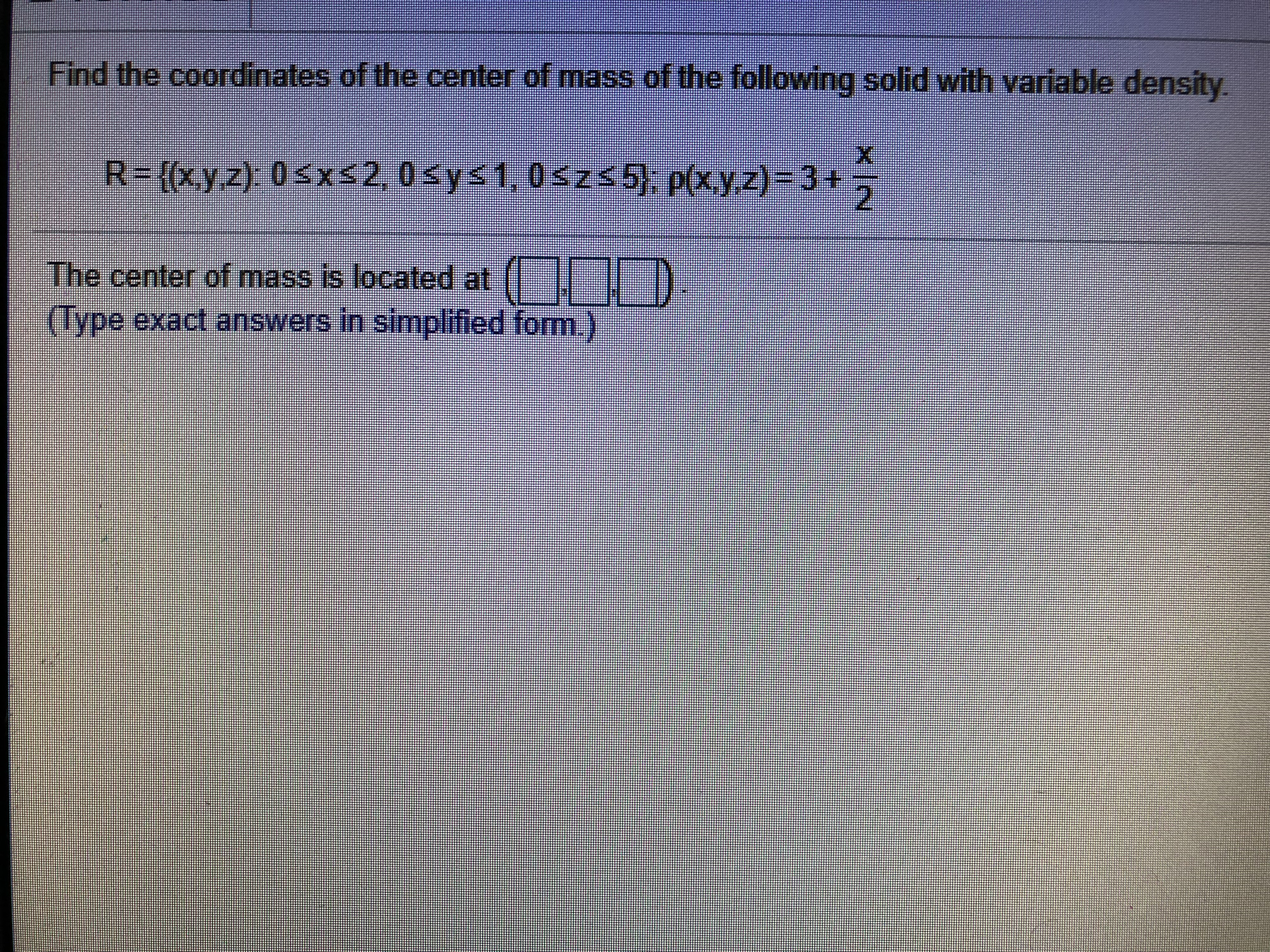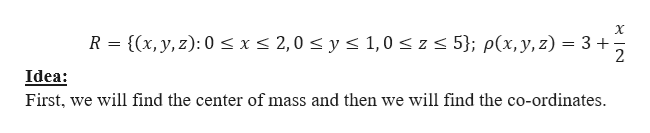# Find the coordinates of the center of mass of the following solid with variable densityXR- (&,y z). 0sxs2,0sys1, 0szs5), p(xyz)=3+2The center of mass is located(Type exact answers in simplified form.

Question
82 viewshelp_outlineImage TranscriptioncloseFind the coordinates of the center of mass of the following solid with variable density X R- (&,y z). 0sxs2,0sys1, 0szs5), p(xyz)=3+ 2 The center of mass is located (Type exact answers in simplified form. fullscreen
check_circle

Step 1

We need to find the co-ordinates of the centre of mass of the solid with variable density.help_outlineImage TranscriptioncloseX R {(x, y, z): 0 < x < 2,0 z < 5}; p(x, y, z) 3 y s 1,0 2 Idea: First, we will find the center of mass and then we will find the co-ordinates fullscreen
Step 2

Now,

Step 3

Now, the co-ordin...

### Want to see the full answer?

See Solution

#### Want to see this answer and more?

Solutions are written by subject experts who are available 24/7. Questions are typically answered within 1 hour.*

See Solution
*Response times may vary by subject and question.
Tagged in

### Other